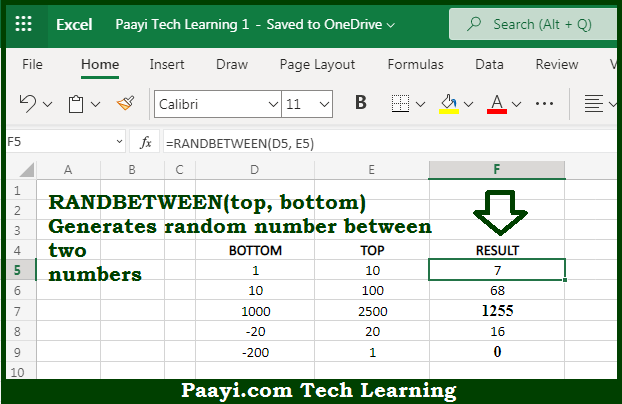# Learn How to Use Microsoft Excel RANDBETWEEN Function

Written by | 0 Comments | 575 Views

In this article, you will learn how to use the Microsoft Excel RANDBETWEEN function and its prime function in Microsoft Excel. You will also get to know the Microsoft Excel RANDBETWEEN function return value and syntax with the help of some examples.

Microsoft Excel RANDBETWEEN Function

The main function of the Microsoft Excel RANDBETWEEN function is to get the random integers between two given values. That implies, with the help of the RANDBETWEEN function you can able to return the random integers between given values. It should be noted that the RANDBETWEEN function recalculates when the worksheet is opened or changed. So, with the help of the RANDBETWEEN function, you can able to get the random integers between two values.

Return Value of RANDBETWEEN Function

The return value will be the integer.

Syntax of RANDBETWEEN Function

=RANDBETWEEN(bottom, top)

Where the arguments:

• bottom: This is the integer representing the lower value of the range.
• top: This is the integer representing the upper value of the range.

## How to Use Microsoft Excel RANDBETWEEN Function?So we know that Microsoft Excel RANDBETWEEN function you can able to get the random integers between two given values. That implies, with the help of the RANDBETWEEN function you can able to return the random integers between given values. It should be noted that the RANDBETWEEN function recalculates when the worksheet is opened or changed. So, with the help of the RANDBETWEEN function, you can able to get the random integers between two values.